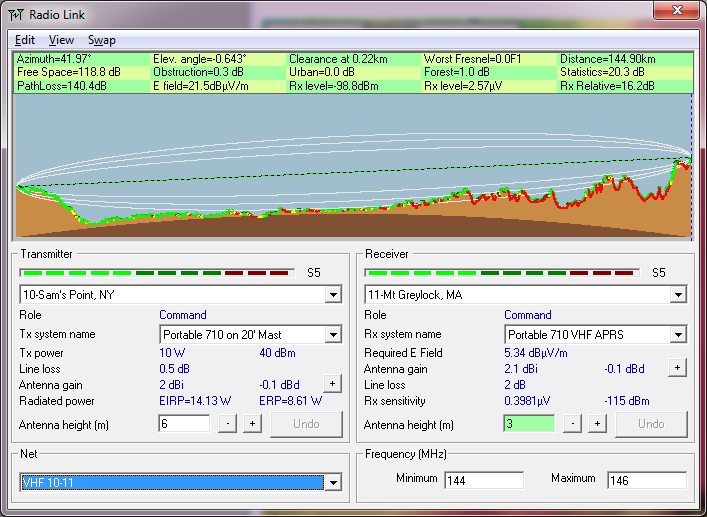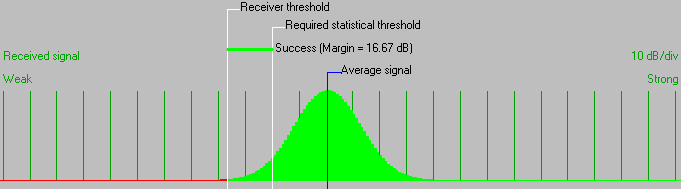• Distance between 10-Sam's Point, NY and 11-Mt Greylock, MA is 144.9 km (90.0 miles)
• True North Azimuth = 41.97°, Magnetic North Azimuth = 54.98°, Elevation angle = -0.6426°
• Terrain elevation variation is 1062.3 m
• Propagation mode is line-of-sight, minimum clearance 0.0F1 at 0.2km
• Average frequency is 145.000 MHz
• Free Space = 118.8 dB, Obstruction = 0.3 dB, Urban = 0.0 dB, Forest = 1.0 dB, Statistics = 20.3 dB
• Total propagation loss is 140.4 dB (interference mode (optimistic))
• System gain from 10-Sam's Point, NY to 11-Mt Greylock, MA is 156.6 dB
• System gain from 11-Mt Greylock, MA to 10-Sam's Point, NY is 156.6 dB
• Worst reception is 16.2 dB over the required signal to meet 95.000% of situations